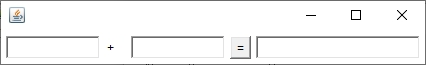﻿ Java窗口精细全方位讲解_java_脚本之家
java# Java窗口精细全方位讲解## 一、新建简单窗口

```import java.awt.*; //包含用于创建用户界面和绘制图形图像的所有类。
```

API下载地址

```import java.awt.*;
public class chuangko{
public static void main(String args[]){
Frame p=new Frame("Hello");//定义窗口名称
p.setSize(500,500);//设置窗口大小（长，宽）
p.setLocation(300,200);//设置窗口左上角点界面位置（长，宽）
p.setBackground(Color.BLUE);//设置窗口内部颜色
p.setVisible(true);//显示窗口
}
}
```ps:是不是感觉也不难哎## 二、编写窗口中的按键

```public class Button
extends Component
implements Accessible
//此类创建一个标签按钮。当按下该按钮时，

```

```import java.awt.*;
public class chuangko{
public static void main(String args[]){
Frame p=new Frame("Hello");
p.setSize(1000,500);
p.setLocation(300,200);
p.setBackground(Color.BLUE);
/*或者：
Button b=new Button("！");
*/
p.setVisible(true);
}
}
```

```import java.awt.*;
public class chuangko{
public static void main(String[] args){
Frame f=new Frame("Hello");
f.setLayout(new GridLayout(2,1));//初始化按键布局样式，两行一列
f.setSize(300,200);
f.setBackground(new Color(200,50,104));//定义窗口背景颜色，括号内代表颜色取值
f.setLocation(500,400);
Panel a=new Panel(new BorderLayout());//定义上半部分
Panel b=new Panel(new BorderLayout());//定义下半部分
Panel a1=new Panel(new GridLayout(2,1));//定义一个值a1，是两行一列
Panel b1=new Panel(new GridLayout(2,2));//定义一个值b1，是两行2列
for(int i=0;i<4;i++)
{
}

f.setVisible(true);//显示
}
}
``````public abstract class Monitor
implements MonitorMBean, MBeanRegistration
//定义所有监视器 MBean 的公共部分。

```

```import java.awt.*;
import java.awt.event.*;
public class jt{
public static void main(String[] args){
Frame f=new  Frame("监听");
Button b=new Button("!!!!!");
Monitor bh=new Monitor();//监听,new谁调用谁
f.pack();//根据按键设置页面合理大小
f.setVisible(true);
}
}
class Monitor implements ActionListener //接口
{
public void actionPerformed(ActionEvent e)//封装
{
System.out.println("ok");//监听表述
}
}
```

## 三、简单的按键运行

### 1.流布局管理器：### 2.静态文本框：

```Label a=new Label(" ");
```

```import java.awt.*;
import java.awt.event.*;
public class jiafa{
public static void main(String[] args){
new TFFrame().launchFrame();
}
}
class TFFrame extends Frame{
TextField tf,tf1,tf2;//在外部定义，否则调用不上！
public void launchFrame()//运行
{
tf=new TextField(10);//允许编辑单行文本的文本组件,设置宽度
tf1=new TextField(10);
tf2=new TextField(20);
Label lblPlus=new Label("+");
//输入静态文本,是一个可在容器中放置文本的组件。一个标签只显示一行只读文本。文本可由应用程序更改，但是用户不能直接对其进行编辑。
Button b=new Button("=");//设置按键
setLayout(new FlowLayout());//使用流布局管理器
pack();
setVisible(true);//显示输入框
}
//声明在class里面,方法外面！！！
private /* 只可以自己访问*/ class MyMonitor implements ActionListener//直接定义内部类,可以直接访问！！！！
{
public void actionPerformed (ActionEvent e)//封装
{
int n1=Integer.parseInt(tf.getText());//将文本框输入值调用Integer
int n2=Integer.parseInt(tf1.getText());
tf2.setText(""+(n1+n2));//定义值的运算
}
}
}
```————————————————————手动分割—————————————————————————————————————————手动分割—————————————————————```this.addWindowListener(
{
public void windowClosing(WindowEvent e)//封装窗口关闭类型
{
setVisible(false);
System.exit(0);//显示关闭
}
}
);
```

## 四、窗口画图ps:是真的真的很简单哎

```public class Color
extends Object
implements Paint, Serializable
//Color 类用于封装默认 sRGB 颜色空间中的颜色，或者用于封装由 ColorSpace 标识的任意颜色空间中的颜色

```
```public abstract class Graphics
extends Object
```

Color类用于设定图像颜色；Graphics类用于设定图像类型；

```public void paint (Graphics g)
{
}
```

```import java.awt.*;
import java.awt.event.*;
public class ht{
public static void main(String[] args){
new PainFrame().launchFrame();
}
}
class PainFrame extends Frame{
public void launchFrame(){
setBounds(200,0,640,480);//定义界面位置及其大小
setVisible(true);
//拿来吧你
(
{
public void windowClosing(WindowEvent e)
{
setVisible(false);
System.exit(0);
}
}
);
}

public void paint(Graphics g)//绘制对象,图像上进行绘制
//Graphics 类是所有图形上下文的抽象基类，允许应用程序在组件（已经在各种设备上实现）以及闭屏图像上进行绘制。
{
Color c=g.getColor();//定义新建颜色
g.setColor(Color.red);//颜色
g.fillOval(50,60,50,50);//定义图像样式、位置及其大小
g.setColor(Color.blue);
g.fillRect(200,360,80,50);
g.setColor(c);//回复现场（习惯）
}
}
```## 五、窗口鼠标响应```构造方法摘要
Point()
在坐标空间的原点 (0,0) 构造并初始化一个点。
Point(int x, int y)
在坐标空间中指定的 (x,y) 位置构造并初始化一个点。
Point(Point p)
构造并初始化一个与指定的 Point 对象具有相同位置的点。

```

```方法摘要
void mouseClicked(MouseEvent e)
鼠标按键在组件上单击（按下并释放）时调用。
void mouseEntered(MouseEvent e)
鼠标进入到组件上时调用。
void mouseExited(MouseEvent e)
鼠标离开组件时调用。
void mousePressed(MouseEvent e)
鼠标按键在组件上按下时调用。
void mouseReleased(MouseEvent e)
鼠标按钮在组件上释放时调用。

```

```import java.util.*;
import java.awt.*;
import java.awt.event.*;
public class shub{
public static void main(String[] args){
new MyFrame("孤寡");//窗口名称

}
}
class MyFrame extends Frame{
ArrayList<Point> points=null;//初始化接口,<Point> 指定只能装入类型
MyFrame(String s){
super(s);
points=new ArrayList<Point> ();//将空间（x，y）放入接口,<Point> 指定只能装入类型
setLayout(null);
setBounds(600,200,520,520);//文本框大小位置
this.setBackground(new Color(255,100,100));
setVisible(true);
/**/  //关闭窗口，写在显示窗口后面！
{
public void windowClosing(WindowEvent e)//封装窗口关闭类型
{
setVisible(false);
System.exit(0);//显示关闭
}
}
);
/**/
}
public void paint(Graphics g)//画图（鼠标点完的样式）
{
Iterator<Point> a=points.iterator();//指定拿出只能是point
while(a.hasNext())//每点一次出一次图案，循环
{
Point p=(Point)a.next();
g.setColor(Color.RED);
g.fillOval(p.x,p.y,10,10);

}

}
}

}
{
public void mousePressed(MouseEvent e)//接收后封装,方法类型在里面
{
MyFrame f=(MyFrame)e.getSource();//拿到MyFrame事件源
f.repaint();//将Frame进行重画,重绘此组件。
/*repaint-update()-paint(),调用顺序(双缓冲)*/
}
}
```## 六、总结```import java.awt.*;
import java.awt.event.*;
public class pf{
public static void main(String[] args){
//new MyFrame("平方");
new TFFrame().launchFrame();
}
}
class TFFrame extends Frame{
TextField n1,n2;
public void launchFrame()
{
//Frame f=new Frame("平方");
n1=new TextField(10);
n2=new TextField(15);
Label f=new Label("平方");
Button b=new Button("=");
setLayout(new FlowLayout());
pack();
setVisible(true);
(
{
public void windowClosing(WindowEvent e)
{
setVisible(false);
System.exit(0);
}
}
);
}
private class MyMonitor implements ActionListener{
public void actionPerformed (ActionEvent e){
int a=Integer.parseInt(n1.getText());
n2.setText(""+(a*a));
}
}
}
```